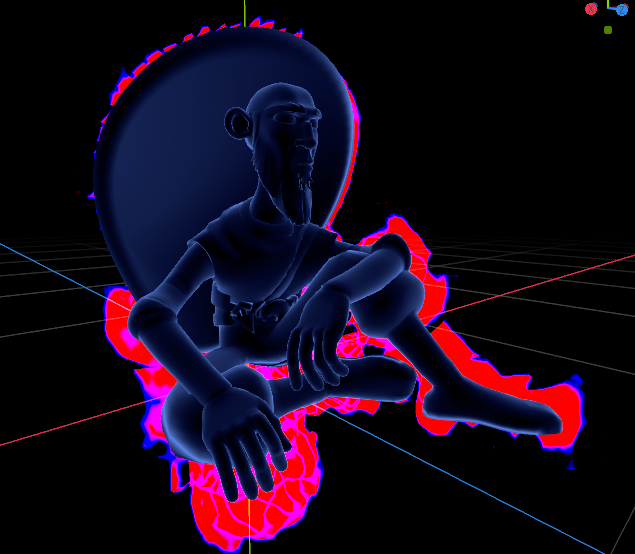# Aura (3D)

This is an attempt to translate from Unity this shader: original, it adds an “Aura” to 3D models, the default parameters are fine but the noise texture needs some adjustments:

Texture properties

Check mark as Seamless
Check as Normalmap
Set Bump Strenght to 32

Noise properties

Set Octaves to 8
Set Period to 32
Set Persistance to 0.75
Set Lacunarity to 2.75

``````shader_type spatial;

uniform sampler2D _NoiseTex;
uniform float _Scale : hint_range(0.0, 0.05) = 0.05;
uniform float _Opacity: hint_range(0.01, 10.0) = 10.0;
uniform float _Edge : hint_range(0.0, 1.0) = 0.1;
uniform vec4 _ColorAura : hint_color = vec4(1.0,0.0,0.0,1.0);
uniform vec4 _ColorRim : hint_color = vec4(0.0,0.0,1.0,1.0);
uniform float _Brightness: hint_range(0.5, 20.0) = 2.0;
uniform float _SpeedX: hint_range(-10.0, 10.0) = 0.0;
uniform float _SpeedY: hint_range(-10.0, 10.0) = 3.0;
uniform float _OffsetFade: hint_range(-10.0, 10.0) = 1.0;
uniform float _GlowBrightness: hint_range(0.01, 30.0) = 3.0;
uniform float _OutlineFixed: hint_range(0.0, 5.0) = 2.0;
uniform float _RimPower2: hint_range(0.01, 10.0) = 6.0;

void vertex()
{
VERTEX	 = (NORMAL * _Edge) + VERTEX;
}
float saturate(float value)
{
return clamp(value,0.0,1.0);
}

void fragment() {
// noise
float speedx = TIME * _SpeedX * 0.005;
float speedy = TIME * _SpeedY * -0.005;
vec4 n = texture(_NoiseTex, vec2(SCREEN_UV.x * _Scale + speedx, SCREEN_UV.y * _Scale + speedy));
// same noise, but bigger
vec4 n2 = texture(_NoiseTex, vec2(SCREEN_UV.x* (_Scale * 0.5) + speedx, SCREEN_UV.y * (_Scale * 0.5) + speedy));
// same but smaller
vec4 n3 = texture(_NoiseTex, vec2(SCREEN_UV.x* (_Scale * 2.0) + speedx, SCREEN_UV.y * (_Scale * 2.0) + speedy));
// combined
float combinedNoise = (n.r * n2.r * 2.0) * n3.r * 2.0;

float rims = pow(saturate(dot(VIEW, NORMAL)), _RimPower2); // calculate inverted rim based on view and normal
vec4 rim = vec4(rims);
rim -= combinedNoise; // subtract noise texture
rim += (rims * _OutlineFixed);
vec4 texturedRim = vec4(saturate(rim.a * _Opacity)); // make a harder edge
vec4 extraRim = (saturate((_Edge + rim.a) * _Opacity) - texturedRim) * _Brightness;// extra edge, subtracting the textured rim
vec4 result = (_ColorAura * texturedRim) + (_ColorRim * extraRim);// combine both with colors

EMISSION = vec3(result.r, result.g, result.b);
ALPHA = result.a;
}
``````The shader code and all code snippets in this post are under CC0 license and can be used freely without the author's permission. Images and videos, and assets depicted in those, do not fall under this license. For more info, see our License terms.Inline FeedbacksNekotoArts
1 year ago

Ayt, time to make JoJo in Godot.Chris
1 year ago

It does not work with my low poly model. It becomes emerged with this aura. Or is it this kind of shader that is applied to a duplicated mesh ?David Ochoa
1 year ago

It is a duplicated mesh (scaled and front culling) I didn’t test it with a low poly model.Dylan
1 year ago

Hi mate I’ve been fiddling with this this afternoon because it’s exactly the effect I was trying to achieve, and I have been running in to one problem I can’t quite figure out: when the camera is viewing the mesh with this shader attached from certain angles, the mesh starts to be culled from the bottom, to the point where if I am viewing the model from below looking up, it will be invisible. What do you think the issue here might be from? Cheersanimanoir
1 year ago

nice my friend